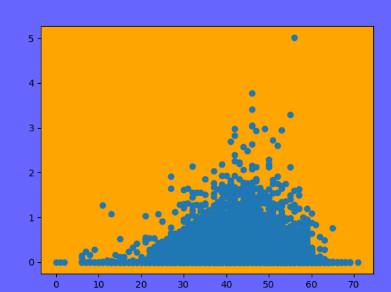# Matplotlib: Change Figure Background Using fig.patch.set_facecolor()

In this tutorial, we will use an example to show you how to change figure background using fig.patch.set_facecolor() in matplotlib.We will use tutorial in

Matplotlib: Create a Plot Using plt.scatter()

to draw a plot.

```PRCP = weather_data['PRCP']
TMAX = weather_data['TMAX']
TMIN = weather_data['TMIN']```

2.Create a figure in matplotlib

`fig = plt.figure()`

3.Set background color for figure

```fig.patch.set_facecolor('blue')
fig.patch.set_alpha(0.6)```

In this code, we use fig.patch.set_facecolor() to set the background color of figure to blue.

Of course, you also can use fig.patch.set_alpha() to set the alpha of the figure.

4.Display plot

```ax = fig.add_subplot(111)
ax.patch.set_facecolor('orange')
ax.patch.set_alpha(1.0)

plt.scatter(TMIN, PRCP)
plt.show()
```

In this code, we also set the background of axes.

Matplotlib: Change Plot Axes Background Using ax.set_facecolor()

Run this code, you will see this plot: